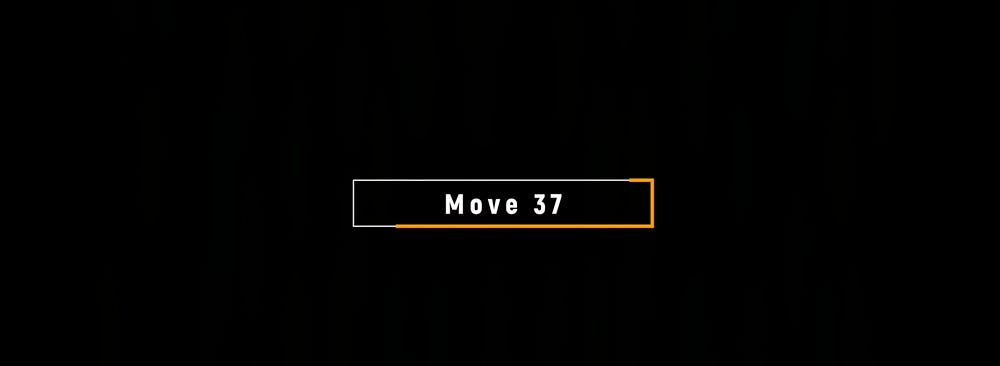01. Reinforcement Learning (Move 37): Introduction

A summary of the concepts discussed in the Introductory lecture for a course from the School of AI called Move 37. The course covers reinforcement learning from the basics up to modern day techniques.

Supervised Learning

How Supervised Machine Learning Works

Step 1: Provide the machine learning algorithm categorised or “labelled” input and output data to learn.

Step 2: Feed the machine new, unlabelled info to see if it tags new data appropriately, if not continue refining the algorithm.

In supervised learning the data already contains the desired results, which is known as the dependent variable.

The objective is to try and find / learn a function that relates the Input Features to the Label.

Types of Problems to which it’s suited

• Classification — Sorting items into categories
• Regression — Identify real values

Unsupervised Learning

How Unsupervised Machine Learning Works

Step 1: Provide the machine learning algorithm categorised, unlabelled input data to see what patterns it finds.

Step 2: Observe and learn from the patterns the machine identifies.

In unsupervised learning there are no clean labels but we still want to derive insights from the data.

Types of problems to which it’s suited

Clustering — Identifying similarities in groups

Anomaly detection — Identifying abnormalities in data (what data doesn’t fit in with the rest)

Typical used to preprocess data in the exploratory analysis phase for supervised learning.

Key differences between supervised and unsupervised learning

Supervised Learning

• Labelled data
• Direct Feedback
• Predict Output

Unsupervised Learning

• Non-labelled data
• No feedback
• Find hidden structure in data

Reinforcement Learning

Scenario: Want to move a product from point A to point B. So many potential problems such as transport breakdowns, bad weather, product destroyed etc.

What kind of learning technique can be used to predict the most optimal route given all the other factors. Potential issues include:

• A highly dynamic learning space which need a product that is highly adaptive to changes.
• No preexisting dataset to learn from.
• Have to learn in real time what works and what doesn’t in a setting that introduces an entirely new dimension time.

Reinforcement learning falls between supervised and unsupervised learning, where we have time delayed labels that are sparse (we don’t get that many). These time delayed labels are known as rewards, in which we use learn how to behave in the environment.

Pure Reinforcement Learning

Define a mathematical framework that encapsulates AI interacting with an environment where time is a dimension and learns through trial and error

Markov chain: Follows a chain of linked events. Which has a set of states and a process that can move successively from one state to another. Each move is a single step and is based on a transition model (T) which defines how to move from one state to the next. The chain is based on a property Markov Property.

The Markov Property states that given the present, the future is conditionally independent of the past. The state in which the process is now, is dependent only on the state, the process was one time step ago.

Markov Chain Model

• Transition Probabilities are edge in the graph
• States of the chain are the nodes

Markov Decision Process

Most common framework for representing the reinforcement learning problem of an agent learning in an environment is called a Markov Decision Process, which is an extension of Markov Chains with the addition of actions which allows choice, rewards and motivation.

Markov Decision Process has 5 components:

• S ={s1,s2,s3} — A set of possible states
• A={a1, a2, a3} — Set of Actions
• Pr(s’|a,s) — Transitions
• β — Starting State Distribution
• r(s,a) — Reward

The transition model returns the probability of reaching the next state if the action is done in a previous state but given S and A the model is conditionally independent of all previous states and actions which is the Markov property. The reward function R returns a real value every time the agent moves from one state to the other. Since we have a reward function we can conclude that some states are more desirable than others, because when the agent moves to these states the agent receives a high reward and there are also cases where an agent receives negative reward and these states are undesirable.

Objective is for the agent to maximise the reward by avoiding negative reward value states and choosing positive value states.

The solution is to find a Policy which selects an action with the highest reward. Agents can try different policies but only one can be considered an optimal policy which gives the best utility.

Key Question (to be answered in further lectures): How does an agent choose the best / optimal policy?

Key takeaways:

1. In reinforcement learning, an AI learns how to optimally interact in a real-time environment using time-delayed labels, called rewards as a signal
2. The Markov Decision Process is a mathematical framework for defining the reinforcement learning problem using states, actions and rewards
3. Through interacting with the environment, an AI will learn a policy which will return an action for a given state with the highest reward

Source: Deep Learning on Medium